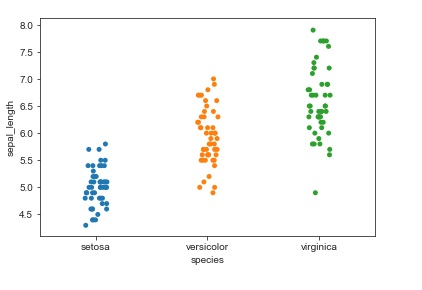# How can Seaborn library be used to display categorical scatter plots in Python?

Seaborn is a library that helps in visualizing data. It comes with customized themes and a high level interface.

General scatter plots, histograms, etc can’t be used when the variables that need to be worked with are categorical in nature. This is when categorical scatterplots need to be used.

Plots such as ‘stripplot’, ‘swarmplot’ are used to work with categorical variables. The ‘stripplot’ function is used when atleast one of the variable is categorical. The data is represented in a sorted manner along one of the axes.

Syntax of stripplot function

seaborn.stripplot(x, y,data,…)

Let us see how ‘stripplot’ function can be used to plot categorical variables in a dataset.

## Example

import pandas as pd
import seaborn as sb
from matplotlib import pyplot as plt
sb.stripplot(x = "species", y = "sepal_length", data = my_df)
plt.show()

## Output## Explanation

• The required packages are imported.
• The input data is ‘iris_data’ which is loaded from the scikit learn library.
• This data is stored in a dataframe.